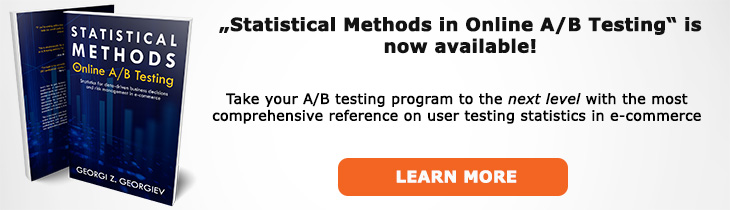# What does "Minimum Detectable Effect" mean?

Definition of Minimum Detectable Effect in the context of A/B testing (online controlled experiments).

## What is a Minimum Detectable Effect?

Aliases: MDE

The minimum detectable effect (MDE) is the effect size which, if it truly exists, can be detected with a given probability with a statistical test of a certain significance level. It is a technical characteristic of the statistical hypothesis test which is fully determined by the choice of the test's sample size and significance threshold. These two parameters fix the power function values and then it is simply a matter of examining it for different values of the power level, beta (see statistical power) to see what effect size can be detected with a given probability.

The higher the power (higher test sensitivity, higher probability to detect as statistically significant, if it truly exists) one wants, the larger the minimum detectable effect becomes assuming the sample size and significance threshold remain unchanged. On the other hand, the larger the sample size, the smaller the MDE becomes at any given power level. The MDE is inversely related to the significance threshold - the lower the p-value becomes, the larger the minimum detectable effect gets.

The minimum detectable effect is closely related to the concept of a minimum effect of interest (MEI). The MEI determines what MDE one wants a given statistical test to have. Examining MDEs produced by different experiment designs is a part of the process of arriving at a proper minimum effect of interest.

Sometimes the term "Minimum detectable effect" (MDE) is used instead of mininmum effect of interest (MEI), but this can be confusing since it refers to a technical characteristic of the statistical test instead of an input parameter for such a test. The MDE wording also confuses practitioners as it hints that true effects below it would not be detected. However, this is not true which can easily be established by examining the power function (which has a value of alpha (α) at the point of the null hypothesis closest to the alternative hypothesis). An alternative term for MDE is MRDE, or minimum reliably detectable effect, which can help avoid such confusion as well as point out that the MDE is always bound to the power level for which it has been calculated.

The term "minimum effect of interest" should be preferred when talking about an input parameter for a test design since it reflects the fact that it is the minimum effect we want to detect reliably and does not carry with it the possibility of confusing it with a minimum effect size which can produce a statistically significant outcome which is a completely different quantity from both MEI and MDE. MDE should be reserved to descriptions of statistical tests which were determined given a certain sample size and significance threshold whereas MEI is to be used when it is an input and refers to an MDE based on which the sample size of a test is to be determined.

## Related A/B Testing terms

Statistical Power

Sample Size

Minimum Effect of Interest

Effect Size

## Articles on Minimum Detectable Effect

Like this glossary entry? For an in-depth and comprehensive reading on A/B testing stats, check out the book "Statistical Methods in Online A/B Testing" by the author of this glossary, Georgi Georgiev.## Glossary Index by Letter

Select a letter to see all A/B testing terms starting with that letter or visit the Glossary homepage to see all.